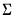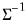Command Reference : Matrix Language Reference

 rmvnorm
Fill matrix with multivariate normals random draws.
Syntax: rmvnorm(x, S[, prefix]))
Syntax: rmvnormc(x, S[, prefix]))
Syntax: rmvnormi(x, S[, prefix]))
Syntax: mvnormci(x, S[, prefix]))
Argument 1: matrix or vector m, sym S
If x doesn't exist, a group object is first created and populated with series named “<prefix>1”, “<prefix>2”, and so on. If omitted, the default prefix is "SER".
If x is a group, the command fills each observation of the contained series with a draw from the distribution.
If x is a vector, the command fills the vector with a single draw from the distribution.
If x is a matrix, the command fills each row of the matrix with a draw from the distribution.
Note that there are actually four distinct commands in this family which correspond to different interpretations for S. These forms are distinguished by the characters in the command name that follow the initial string “rmvnorm”:

 “” Supply, the covariance matrix of distribution. “c” Supply the Cholesky decomposition of. This form is more efficient when performing multiple draws from the same distribution (compute the Cholesky once, but sample many times). “i “ Supply. This form is more efficient than explicitly invertingto supply. “ic” Supply the Cholesky decomposition of. This form combines the efficiencies of the above forms.
Examples
sym a = @inner(@mnrnd(100, 10))
matrix(1000, 10) x
rmvnorm(x, a)
rmvnormc(x, @cholesky(a))
rmvnormi(x, @inverse(a))
rmvnormic(x, @cholesky(@inverse(a))
Cross-references